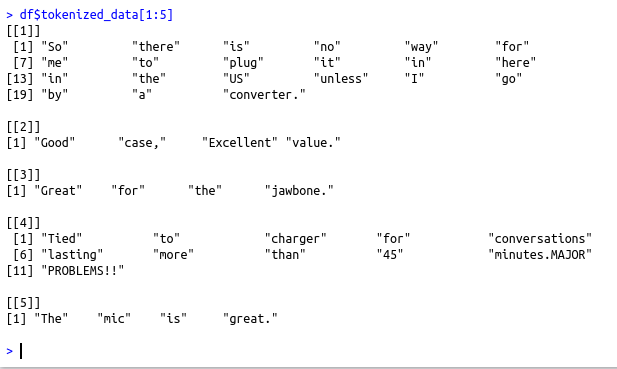• #5, First Floor, 4th Street Dr. Subbarayan Nagar Kodambakkam, Chennai-600 024 Landmark : Samiyar Madam
• pro@slogix.in
• +91- 81240 01111

How to remove stop words using regular expression in R?

Description

To remove stop words using regular expression in R

Functions Used

strsplit(data,” “) – To Tokenize the text by splitting it using space
grepl(stpwrd,data) – To get the logical values of the data with respect to the stpwrd
evaluate() – To evaluate the loss and metrics

Process

Transform the data set into a format as required(Skip this step if it is in proper format)

Tokenize the text by splitting the text using the space character

Check the text data with each stop words and remove it

Update the text data with processed data(stop words removed data)

Sapmle Code

library(stopwords)
data data1=(strsplit(data\$text,”\n”))
data2=unlist(data1[])
data2
data3=strsplit(data2,”\t”)
data4=unlist(data3)
i=0
j=0
k=0
text=c()
pol=c()
for (i in (1:length(data4)))
{
if(i%%2!=0)
{
j=j+1
text[j]=data4[i]
}else
{
k=k+1
pol[k]=data4[i]
}
}
df=data.frame(text=text,polarity=pol,stringsAsFactors = FALSE)
#Split the data with the space character to tokenize each word
v1=strsplit(df\$text,” “)
#Now add the tokenized data as a new column to the existing data frame
df\$tokenized_data df\$tokenized_data[1:10]
#Load the stop words from the package “stopwords”
stp_wrds=stopwords(“en”)
#Paste the caret(^) symbol at the beginning and dollar(\$) symbol at the end to perform exact matching
stp=paste(“^”,stp_wrds,”\$”,sep=””)
#For each stop words check the text data that is converted to lower case
for (i in (1:length(stp)))
{
for (j in (1:length(df\$tokenized_data)))
{
logi=grepl(stp[i],tolower(df\$tokenized_data[[j]]))
logi=!logi
df\$tokenized_data[[j]]=df\$tokenized_data[[j]][logi]
}
}
df\$tokenized_data[1:10]

Screenshots